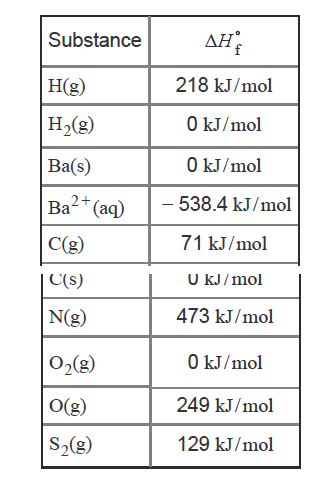# Problem: What is the balanced chemical equation for the reaction used to calculate ΔH°f of BaCO3(s)?If fractional coefficients are required, enter them as a fraction (i.e. 1/3). Indicate the physical states using the abbreviation (s), (l), or (g) for solid, liquid, or gas, respectively without indicating allotropes. Use (aq) for aqueous solution.Express your answer as a chemical equation.The standard enthalpy of formation (ΔH°f ) is the enthalpy change that occurs when exactly 1 mol of a compound is formed from its constituent elements under standard conditions. The standard conditions are 1 atm pressure, a temperature of 25°C , and all the species present at a concentration of 1 M. A "standard enthalpies of formation table" containing ΔH°f values might look something like this:

###### FREE Expert Solution
94% (264 ratings)View Complete Written Solution
###### Problem Details

What is the balanced chemical equation for the reaction used to calculate ΔH°f of BaCO3(s)?

If fractional coefficients are required, enter them as a fraction (i.e. 1/3). Indicate the physical states using the abbreviation (s), (l), or (g) for solid, liquid, or gas, respectively without indicating allotropes. Use (aq) for aqueous solution.